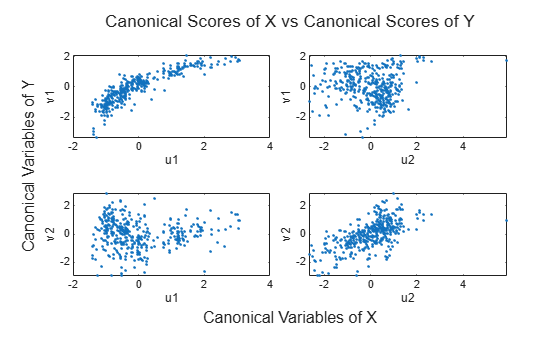# canoncorr

Canonical correlation

## Syntax

``[A,B] = canoncorr(X,Y)``
``[A,B,r] = canoncorr(X,Y)``
``[A,B,r,U,V] = canoncorr(X,Y)``
``[A,B,r,U,V,stats] = canoncorr(X,Y)``

## Description

````[A,B] = canoncorr(X,Y)` computes the sample canonical coefficients for the data matrices `X` and `Y`.```
````[A,B,r] = canoncorr(X,Y)` also returns `r`, a vector of the sample canonical correlations.```

example

````[A,B,r,U,V] = canoncorr(X,Y)` also returns `U` and `V`, matrices of the canonical scores for `X` and `Y`, respectively.```
````[A,B,r,U,V,stats] = canoncorr(X,Y)` also returns `stats`, a structure containing information related to testing the sequence of hypotheses that the remaining correlations are all zero.```

## Examples

collapse all

Perform canonical correlation analysis for a sample data set.

The data set `carbig` contains measurements for 406 cars from the years 1970 to 1982.

```load carbig; data = [Displacement Horsepower Weight Acceleration MPG];```

Define X as the matrix of displacement, horsepower, and weight observations, and `Y` as the matrix of acceleration and MPG observations. Omit rows with insufficient data.

```nans = sum(isnan(data),2) > 0; X = data(~nans,1:3); Y = data(~nans,4:5);```

Compute the sample canonical correlation.

`[A,B,r,U,V] = canoncorr(X,Y);`

View the output of `A` to determine the linear combinations of displacement, horsepower, and weight that make up the canonical variables of `X`.

`A`
```A = 3×2 0.0025 0.0048 0.0202 0.0409 -0.0000 -0.0027 ```

`A(3,1)` is displayed as `—0.000` because it is very small. Display `A(3,1)` separately.

`A(3,1)`
```ans = -2.4737e-05 ```

The first canonical variable of `X` is `u1 = 0.0025*Disp + 0.0202*HP — 0.000025*Wgt`.

The second canonical variable of `X` is `u2 = 0.0048*Disp + 0.0409*HP — 0.0027*Wgt`.

View the output of B to determine the linear combinations of acceleration and MPG that make up the canonical variables of `Y`.

`B`
```B = 2×2 -0.1666 -0.3637 -0.0916 0.1078 ```

The first canonical variable of `Y` is `v1 = ``—``0.1666*Accel — 0.0916*MPG`.

The second canonical variable of `Y` is `v2 = —0.3637*Accel + 0.1078*MPG`.

Plot the scores of the canonical variables of `X` and `Y` against each other.

```t = tiledlayout(2,2); title(t,'Canonical Scores of X vs Canonical Scores of Y') xlabel(t,'Canonical Variables of X') ylabel(t,'Canonical Variables of Y') t.TileSpacing = 'compact'; nexttile plot(U(:,1),V(:,1),'.') xlabel('u1') ylabel('v1') nexttile plot(U(:,2),V(:,1),'.') xlabel('u2') ylabel('v1') nexttile plot(U(:,1),V(:,2),'.') xlabel('u1') ylabel('v2') nexttile plot(U(:,2),V(:,2),'.') xlabel('u2') ylabel('v2')```The pairs of canonical variables $\left\{{u}_{i},{v}_{i}\right\}$ are ordered from the strongest to weakest correlation, with all other pairs independent.

Return the correlation coefficient of the variables `u1` and `v1`.

`r(1)`
```ans = 0.8782 ```

## Input Arguments

collapse all

Input matrix, specified as an n-by-d1 matrix. The rows of `X` correspond to observations, and the columns correspond to variables.

Data Types: `single` | `double`

Input matrix, specified as an n-by-d2 matrix where `X` is an n-by-d1 matrix. The rows of `Y` correspond to observations, and the columns correspond to variables.

Data Types: `single` | `double`

## Output Arguments

collapse all

Sample canonical coefficients for the variables in `X`, returned as a d1-by-d matrix, where d = min(rank(X),rank(Y)).

The jth column of `A` contains the linear combination of variables that makes up the jth canonical variable for `X`.

If `X` is less than full rank, `canoncorr` gives a warning and returns zeros in the rows of `A` corresponding to dependent columns of `X`.

Sample canonical coefficients for the variables in `Y`, returned as a d2-by-d matrix, where d = min(rank(X),rank(Y)).

The jth column of `B` contains the linear combination of variables that makes up the jth canonical variable for `Y`.

If `Y` is less than full rank, `canoncorr` gives a warning and returns zeros in the rows of `B` corresponding to dependent columns of `Y`.

Sample canonical correlations, returned as a 1-by-d vector, where d = min(rank(X),rank(Y)).

The jth element of `r` is the correlation between the jth columns of `U` and `V`.

Canonical scores for the variables in `X`, returned as an n-by-d matrix, where `X` is an n-by-d1 matrix and d = min(rank(X),rank(Y)).

Canonical scores for the variables in `Y`, returned as an n-by-d matrix, where `Y` is an n-by-d2 matrix and d = min(rank(X),rank(Y)).

Hypothesis test information, returned as a structure. This information relates to the sequence of d null hypotheses ${H}_{0}^{\left(k\right)}$ that the (k+1)st through dth correlations are all zero for k=1,…,d-1, and d = min(rank(X),rank(Y)).

The fields of `stats` are 1-by-d vectors with elements corresponding to the values of k.

FieldDescription
`Wilks`

Wilks' lambda (likelihood ratio) statistic

`df1`

Degrees of freedom for the chi-squared statistic, and the numerator degrees of freedom for the F statistic

`df2`

Denominator degrees of freedom for the F statistic

`F`

Rao's approximate F statistic for ${H}_{0}^{\left(k\right)}$

`pF`

Right-tail significance level for `F`

`chisq`

Bartlett's approximate chi-squared statistic for ${H}_{0}^{\left(k\right)}$ with Lawley's modification

`pChisq`

Right-tail significance level for `chisq`

`stats` has two other fields (`dfe` and `p`), which are equal to `df1` and `pChisq`, respectively, and exist for historical reasons.

Data Types: `struct`

collapse all

### Canonical Correlation Analysis

The canonical scores of the data matrices X and Y are defined as

`$\begin{array}{c}{U}_{i}=X{a}_{i}\\ {V}_{i}=Y{b}_{i}\end{array}$`

where ai and bi maximize the Pearson correlation coefficient ρ(Ui,Vi) subject to being uncorrelated to all previous canonical scores and scaled so that Ui and Vi have zero mean and unit variance.

The canonical coefficients of X and Y are the matrices A and B with columns ai and bi, respectively.

The canonical variables of X and Y are the linear combinations of the columns of X and Y given by the canonical coefficients in A and B respectively.

The canonical correlations are the values ρ(Ui,Vi) measuring the correlation of each pair of canonical variables of X and Y.

## Algorithms

`canoncorr` computes `A`, `B`, and `r` using `qr` and `svd`. `canoncorr` computes `U` and `V` as `U = (X—mean(X))*A` and ```V = (Y—mean(Y))*B```.

 Krzanowski, W. J. Principles of Multivariate Analysis: A User's Perspective. New York: Oxford University Press, 1988.

 Seber, G. A. F. Multivariate Observations. Hoboken, NJ: John Wiley & Sons, Inc., 1984.